GFG App
Open AppBrowser
Continue

# Control Table UI Component Appearance and Behavior in MATLAB

In this article, we shall discuss how to create UI tables in MATLAB and control their behaviors and appearance by manipulating some basic properties and visuals. UI tables in MATLAB are the graphical form of tabular data. The UI tables can be created using the uitable function.

### Syntax

tab = uitable(…parameters…)

The uitable without any parameters generates an empty table. Different parameters could be passed to the same for data modification. We shall not discuss creating UI tables in depth as it is not in this article’s scope.

## Creating a Basic UI Table

We can create a UI table by passing a prepared data to a uitable component.

Example 1:

## Matlab

 `uif = uifigure; ` ` `  `age = [32 ;23; 15; 17; 19]; ` `names = {``'Howard'``;``'Harry'``; ``'Mark'``; ``'Nik'``; ``'Mike'``}; ` `gen = {``'M'`  `;``'M'` `; ``'M'``; ``'F'``; ``'M'``}; ` `tab = table(age,names,gen) ` ` `  `tab = uitable(uif,``'Data'``,tab); `

In this example, we create a uifigure component which is the only way of converting a table to a uitable in MATLAB R2018a onwards, the same is explained in detail in the following section. Then, we create a table with three variables. After that, the uitable function is called with the uif uifigure component and tab as the ‘Data’ component.

Output: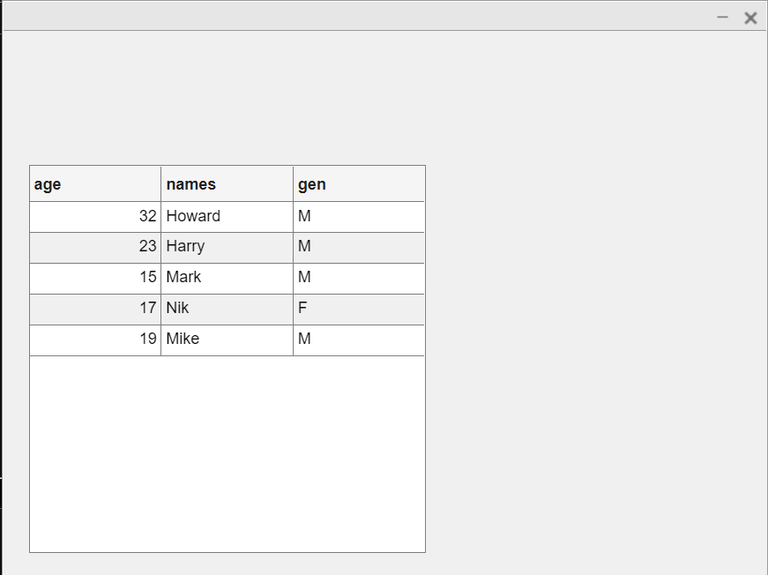This is an example of a simple uitable. We shall use this same table in the following sections to understand the properties of UI tables.

## UI Tables in a UI Figure

By default, a new figure is generated by MATLAB for to create a UI table (without any parent figure).

Example 2:

## Matlab

 `% MATLAB Code ` `tab = uitable;`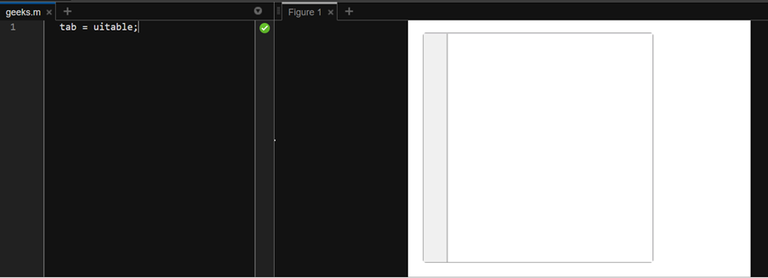However, this is not a UI figure component and thus, we cannot add tabular data to such a UI table. The solution for this problem is creating the UI table within a UI figure component. The same can be done by passing the uifigure name to the uitable function.

## Matlab

 `% MATLAB Code ` `fig = uifigure; ` `tab = uitable(fig);`

In this case, we get a UI figure, which could be developed into an application. The UI table is created inside this UI figure.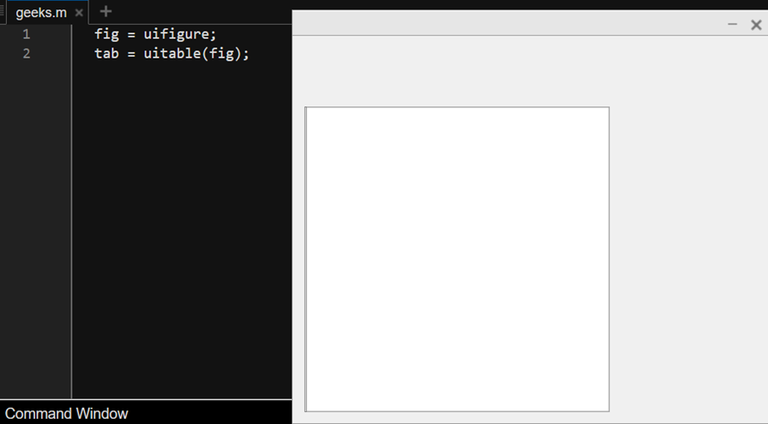As for adding tabular data to this kind of uitable, we have seen the same in previous section.

## Column-Width of UI tables

We can modify the column width of uitables in the table definition itself with the following.

Syntax

tab = uitable(uifigure, ‘ColumnWidth’, {<options>}, ‘Data’, <data>)

The ColumnWidth could have following four values:

1. ‘auto’ – In this case, MATLAB decides the column width automatically, based on factors such as Column name, longest element, etc. This is the default option.
2. ‘fit’ – This option is only available when a uifigure parent is used. This commands the uitable to fit the columns within the restrictions of the uifigure.
3. Ratios – This option for uifigure tables as well. This allows to create columns with widths in ration to each other. The base value is ‘1x’. Its twice would be ‘2x’, and so on.
4. Pixel values – We can pass fixed values for column width in pixel units.

Example 3:

## Matlab

 `% MATLAB Code ` `uif = uifigure; ` ` `  `age = [32 ;23; 15; 17; 19]; ` `names = {``'Howard'``;``'Harry'``; ``'Mark'``; ``'Nik'``; ``'Mike'``}; ` `gen = {``'M'`  `;``'M'` `; ``'M'``; ``'F'``; ``'M'``}; ` `tab = table(age,names,gen); ` ` `  `tab = uitable(uif,``'ColumnWidth'``,{29,``'fit'``,39},``'Data'``,tab);`

Here, we change the width of all three columns from ‘auto’ to 29px, ‘fit’, 39px respectively. The same can be seen below.

Output: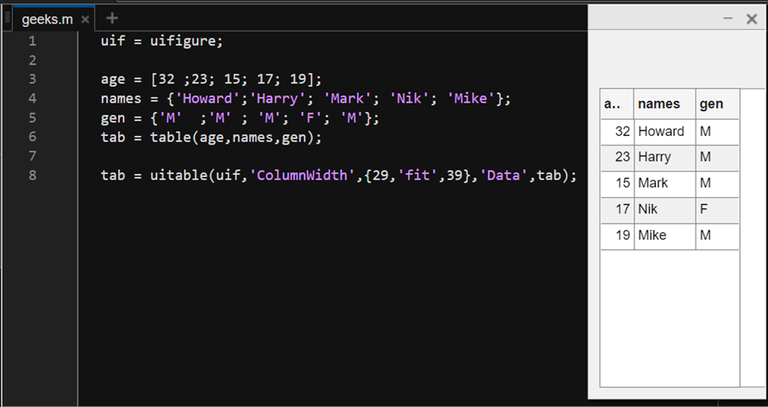Now, let us see an example where we use the ratio widths.

Example 4:

## Matlab

 `% MATLAB Code ` `uif = uifigure; ` ` `  `age = [32 ;23; 15; 17; 19]; ` `names = {``'Howard'``;``'Harry'``; ``'Mark'``; ``'Nik'``; ``'Mike'``}; ` `gen = {``'M'`  `;``'M'` `; ``'M'``; ``'F'``; ``'M'``}; ` `tab = table(age,names,gen); ` ` `  `tab = uitable(uif,``'ColumnWidth'``,{``'1x'``,``'fit'``,``'1x'``},``'Data'``,tab);`

Output: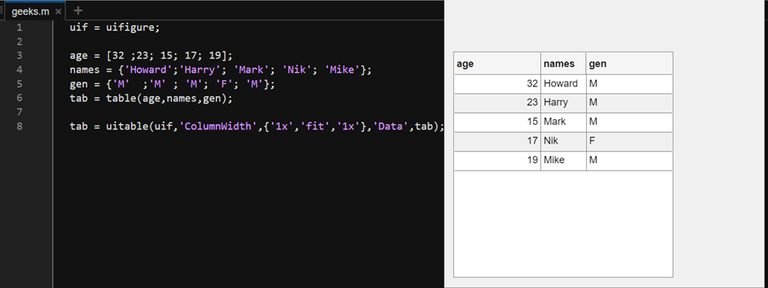As it can be seen, the size of ‘fit’ is much less than ‘auto’, which is the case by definition. Another thing to note down is that the width of ‘auto’ changes with window width however, the width of ‘fit’ does not change even on changing window width.

## Fonts in UI tables

There are various options available for changing the font appearance in a UI table. Discussing them all is not feasible here; therefore, we shall explain the more important ones such as font weight and font angle.

### Font weight

Font weight has 2 values, ‘normal’ and ‘bold’. Both have the same meaning as used in ordinary typing. The default option is ‘normal’; to change the weight to ‘bold’ one needs to use the ‘FontWeight’ option and give its value as ‘bold’.

Example 5:

## Matlab

 `% Code ` `uif = uifigure; ` ` `  `age = [32 ;23; 15; 17; 19]; ` `names = {``'Howard'``;``'Harry'``; ``'Mark'``; ``'Nik'``; ``'Mike'``}; ` `gen = {``'M'`  `;``'M'` `; ``'M'``; ``'F'``; ``'M'``}; ` `tab = table(age,names,gen); ` ` `  `tab = uitable(uif,``'FontWeight'``,``'bold'``,``'Data'``,tab);`

Output: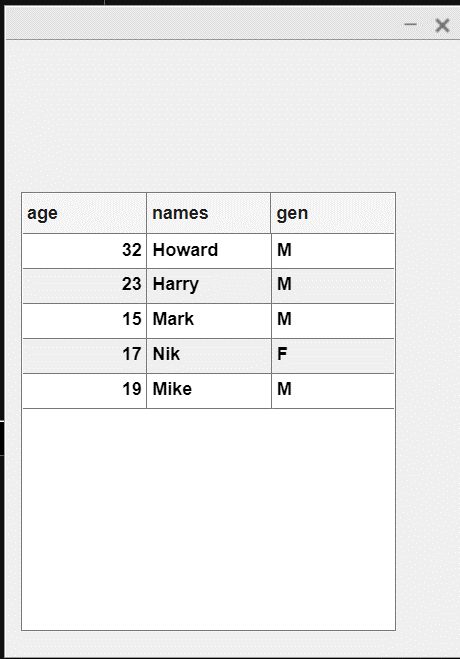As it can be seen, all the fonts are bold now.

### Font Angle

This option is generally used to turn the ‘italic’ font mode on or off. One simply has to use the FontAngle option and pass the argument as ‘italic’. This changes all the font to italic.

Example 7:

## Matlab

 `% Code ` `uif = uifigure; ` ` `  `age = [32 ;23; 15; 17; 19]; ` `names = {``'Howard'``;``'Harry'``; ``'Mark'``; ``'Nik'``; ``'Mike'``}; ` `gen = {``'M'`  `;``'M'` `; ``'M'``; ``'F'``; ``'M'``}; ` `tab = table(age,names,gen); ` ` `  `tab = uitable(uif,``'FontAngle'``,``'italic'``,``'Data'``,tab);`

Output: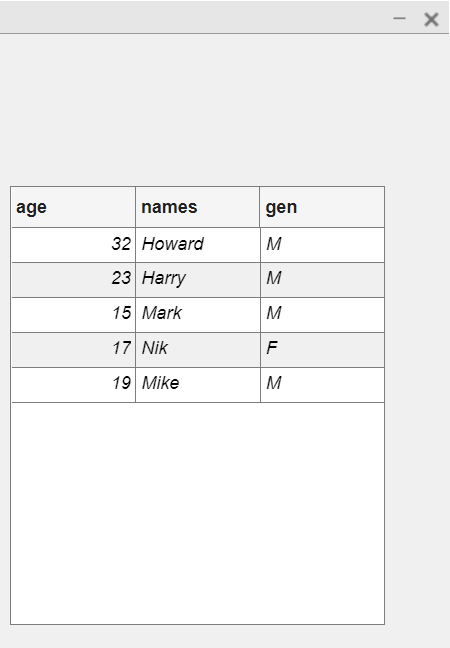## Colors in UI tables

There are two color options available with UI tables in MATLAB, foreground and background. We shall see them both in the following example.

We can change the background and foreground colors by using the ‘BackGround’ and ‘ForeGround’ options respectively and then, passing their respective RGB values following them both. The background changes the color of cells whereas the foreground changes the color of font. See the following example for better understanding.

Example 8:

## Matlab

 `% Code ` `uif = uifigure; ` ` `  `age = [32 ;23; 15; 17; 19]; ` `names = {``'Howard'``;``'Harry'``; ``'Mark'``; ``'Nik'``; ``'Mike'``}; ` `gen = {``'M'`  `;``'M'` `; ``'M'``; ``'F'``; ``'M'``}; ` `tab = table(age,names,gen); ` ` `  `tab = uitable(uif,``'BackgroundColor'``,[1 1 0],``'ForegroundColor'``,[0 0.4470 0.7410],``'Data'``,tab);`

Here, we change the background to yellow and the foreground (font color) to navy blue color using their respective RGB values.

Output: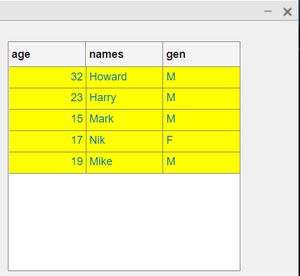As it can be seen, the cell color is yellow, and the font color is a shade of blue.

My Personal Notes arrow_drop_up